# Test: Absolute Equations- 2

## 25 Questions MCQ Test Quantitative Aptitude for GMAT | Test: Absolute Equations- 2

Description
Attempt Test: Absolute Equations- 2 | 25 questions in 42 minutes | Mock test for Quant preparation | Free important questions MCQ to study Quantitative Aptitude for GMAT for Quant Exam | Download free PDF with solutions
QUESTION: 1

### If x2 < 25, which of the following expressions must be true? I.|x-3| < 10 II.|2x – 3| < 8 III. |2x2| < 42

Solution:

Given

• x2 < 25

To Find: The expressions in the option that are always true?

Approach

1. We will convert the expression x2 < 25 to the form (x+a)(x-a) < 0 and find the range of values of x using the wavy line method.
2. Then we will analyse the expressions given in the options to see which of them are always true for the values of x found in (1)

Working Out

1. x2 < 25
1. x2 - 25 < 0
2. x2−52<0
3. (x+5)(x−5)<0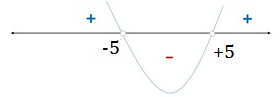1. Hence, -5 < x < 5

1. Evaluating options
1. |x-3| < 10.

• The above inequality refers to all values of x that are at a distance of less than 10 units from 3 on the number line
• -7 < x < 13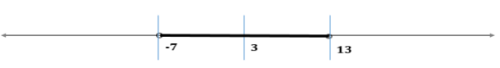i. The inequality can also be solve algebraically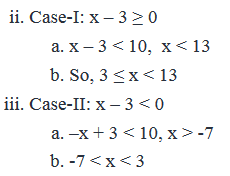iv. Combining the cases above, we can write -7 < x < 13

v. The range -7 < x < 13, covers all the possible values of -5 < x < 5

vi. Hence, this expression is always true

II. |2x – 3| < 8.

1. The inequality can be simplified to |x-1.5| < 4
2. The above inequality refers to all values of x that at a distance of less than 4 units from 1.5 on the number line.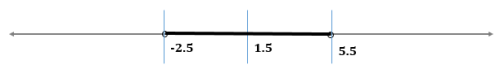3. The inequality can also be solved algebraically as below:
4. Case-I: 2x – 3 ≥ 0
1. 2x – 3 < 8, x < 5.5
2. 1.5 ≤ x < 5.5
5. Case-II: 2x – 3 < 0
1. -2x + 3 < 8, x > -2.5
2. -2.5 < x < 1.5
6. Combining the two cases above, we have -2.5 < x < 5.5
7. As x can take any value between -5 and +5, the above range -2.5 < x < 5.5 does not cover all the possible values of x.
8. Hence, this expression is not always true.

III. |2x2| < 42

1. In this expression, we can see that x2, being the square of a number, must be non-negative (irrespective of the value of x). So, we can write:x2≥0. Therefore, 2x2≥0
2. So, we can write 2x2<42
3. x2 - 21 <0
4. (x+ √21) (x-√21) < 0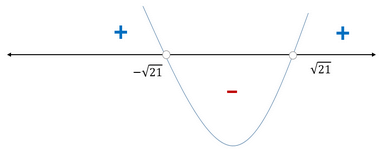5. So, -√21 < x < √21.

6. As x can take any value such that -5 < x < 5 (-√25 < x < √25) , the above range -√21 < x < √21 does not cover all the possible values of x.(For example √24, √23 etc.)

7. Hence, this expression is not always true.

3. So, only expression I is always true

QUESTION: 2

### If p and q are two integers on the number line such that q is on the right hand side of 0 and p is on the right hand side of q at a distance from q that is three times the distance between 0 and q, then what is the least possible distance on the number line between the number -16 and the product of p and q?

Solution:

Given:

• p and q are positive integers as below: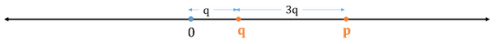To find: The least possible distance between -16 and pq

Approach:

1. Our first step will be to determine the expression for the distance between -16 and pq
1. The distance between -16 and 0 = 16 units
2. The distance between 0 and pq = pq units
3. Since both p and q are positive, pq will be positive as well
4. Therefore, the distance between -16 (which is on left hand side of 0) and pq (which is on the right hand side of 0) will be 16 plus pq, that is,

Distance between -16 and pq = 16 + pq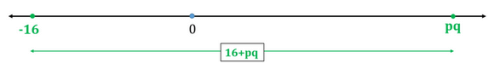2.  In the expression 16 + pq, 16 is constant. So, the value of this expression will be minimum when pq is minimum

3.  To find the minimum value of pq, we will need to simplify the term pq

1. pq = (Value of p)*(Value of q)
• Value of p = Distance of p from 0 on the number line = q + 3q  = 4q
2. So, pq = (4q)*q = 4q2
3. So, to answer the question, we need to find the minimum value of 4q2

4. To find the minimum value of 4q2, we will apply the constraint that q is a positive integer

Working Out:

• The minimum value of 4q2 happens when the value of q2 is minimum
• Value of q2 will be minimum when q is minimum
• Since q is a positive integer, minimum value of q is 1
• Thus, minimum value of 4q2 = 4(1)2 = 4
• And, minimum value of (16 + 4q2) = 16 + 4 = 20

Thus, the correct answer is Option E

QUESTION: 3

### Seven water stations were set up at regular intervals along the 42-kilometer route of a marathon, with the last water station at the finish point. If James maintained a uniform running speed throughout the marathon, did not turn back at any time and reached the finish line, in how much time did he run the marathon? (1) 40 minutes after the start of the marathon, James was 4 kilometers away from the second water station (2) 20 minutes before reaching the finish point, James was 20 kilometers away from the third water station

Solution:

Steps 1 & 2: Understand Question and Draw Inferences

Given:  The 7 water stations are set up at REGULAR intervals

• Let’s mark the Starting Point of the marathon as Zero
• The 7 water stations are then situated as under: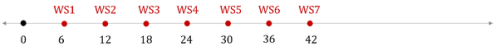• James:
• Total distance run = 42 km
• Uniform speed
• No turning back

To Find: Time taken by James to run 42 km

• To find Time, we need to find his running speed

Step 3: Analyze Statement 1 independently

(1) 40 minutes after the start of the marathon, James was 4 kilometers away from the second water station

• 2nd water station = 12 km from Starting Point
• For Time = 40 min, Distance run =
• (12 + 4 =) 16 km OR
• (12 – 4 = ) 8 km
• So running speed =
• 16/40 km per min OR
• 8/40 km per min

Since we don’t get a unique value of running speed, Statement 1 is not sufficient to get a unique answer

Step 4: Analyze Statement 2 independently

(2) 20 minutes before reaching the finish point, James was 20 kilometers away from the third water station

• 3rd water station = 18 km from Starting Point
• Position 20 minutes before Reaching Finish Point =
• (18 - 20 =) -2 km OR
• (18 + 20 = ) 38 km

• James never turned back (given)
• So, he could not be at position -2 km

• So, James’ position 20 minutes before Reaching Finish Point = 38 km
• Distance run in last 20 min = (42 – 38) km = 4 km
• Speed in last 20 min = 4/20 km per min
• (Since his speed is uniform) James’ Speed throughout the run = His Speed in last 20 min = 4/20  km per min

Since we now know his speed, we can find the time he took to run the entire marathon.

Sufficient

(Alternate analysis once it is determined that Distance run in last 20 min = 4 km:

Time taken to run 4 km = 20 min

Time taken to run 42 km = 20/4 ∗ 42  min

This method too is valid only under the condition of uniform speed)

Step 5: Analyze Both Statements Together (if needed)

Since we arrived at a unique answer in Step 4, this step is not required

QUESTION: 4

If x and y are non-zero numbers and the value of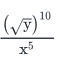is -1, which of the following expressions must be true?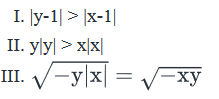Solution:

Given: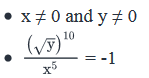• √y is positive. Therefore,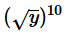is also positive
• Since the right hand side of the above equation is negative,the left hand side must be negative as well.
• So it follows that it is x5 that is negative.
• Since x5 has the same positive – negative nature as x, it means that x is negative.
• To find: Which of the given three expressions must be true ?

Approach:

• We’ll first simplify the given equation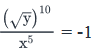• Then, we’ll evaluate the 3 expressions one by one to see.

Working Out: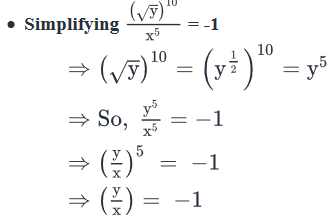• As inferred above, y must be positive and x is negative.
• Therefore, x = -y
• (For example, if y = 3 then x = -3)
• Since y is positive, |y| = y
• Since x is negative, |x| = -x = y
• Thus, |x| = |y| = y
• Evaluating Expression I
• Expression I says that |y-1| > |x-1|
• Now, |y-1| represents the distance of y from 1 on the number line.
• And, |x-1| represents the distance of x from 1 on the number line.
• Remember that y is positive and x is negative and equal to –y.
• Since y’s distance from 1 is the point of consideration in this expression, there are 2 possibilities:
• Case 1: y is a positive number less than or equal to 1
• Case 2: y is a positive number greater than 1
• Let’s represent the distance of y and x from 1 on the number line in both cases.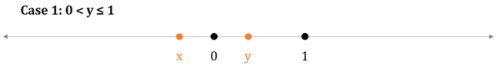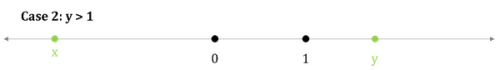• We observe that in both Case 1 and Case 2, |y-1| is lesser than |x-1|
• So, Expression I is definitely not true.
• Evaluating Expression II
• Expression II says that y|y|>x|x|
• Since y is positive, the left hand side of the above expression is always positive. Since x is negative, the right hand side is always negative.
• We know that a Positive value is always greater than a negative value
• So, for all values of x and y, Expression II must be true.
• Evaluating Expression III
• Expression III says that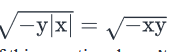• Now the left hand side of this equation doesn’t give a real number. This is because:
• y is positive
• Therefore, -y is negative
• |x| is positive
• So, the product –y|x| is negative
•  Since the value under the square root is negative, this square root will not be a real number
• The Right hand side of the expression, on the other hand, gives a real number
• Since a real number cannot be equal to a number that is not real, Expression III is definitely not true.
• We observe that of the 3 expressions, only Expression II is true.
• Looking at the answer choices, we see that the correct answer is Option B

QUESTION: 5

While driving in one direction on a straight highway, James moves past three inns - Bethlem Inn, The Antimone and The Soliloquy, not necessarily in that order, and then stops at Stoby’s Restaurant for lunch. If the distance between Bethlem Inn and Stoby’s Restaurant is 90 kilometres, what is the distance between The Antimone and Stoby’s Restaurant?

(1) The distance between Bethlem Inn and The Soliloquy is 50 kilometres

(2) The distance between The Antimone and The Soliloquy is 40 kilometres

Solution:

Steps 1 & 2: Understand Question and Draw Inferences

Given:

• Let the alphabets A, B, S, T represent Antimone, Bethlem, Soliloquy and Stoby’s respectively
• BT = 90 where B is on the left of T on the number line (because he passed by B and then reached T)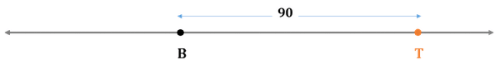To find: AT = ?

• A is on the left of T on the number line (because he passed by A and then reached T)

Step 3: Analyze Statement 1 independently

• The distance between Bethlem Inn and The Soliloquy is 50 kilometres
• This Statement doesn’t give us any clue about the value of AT

So, this statement is clearly not sufficient.

Step 4: Analyze Statement 2 independently

• The distance between The Antimone and The Soliloquy is 40 kilometres
• That is, AS = 40 kilometres
• However, we don’t know the distance ST
• So, we cannot use the fact given in Statement 2 to find the value of AT

Statement 2 is not sufficient

Step 5: Analyze Both Statements Together (if needed)

• From Statement 1: BS = 50
• Note that we do not know the order in which James crossed A, B and S
• So, we have to consider 2 cases as shown below: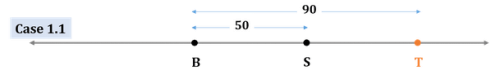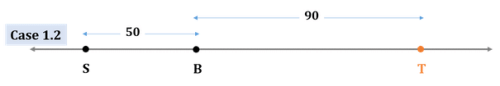• Thus, ST =
• Either 90 – 50 = 40
• Or 90 + 50 = 140

• From Statement 2: AS = 40
• Since we do not know the order in which James crossed A, B and C, we have to consider 2 cases as shown below: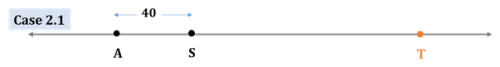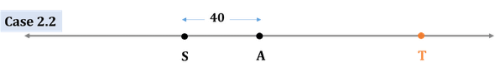• Thus, AT =
• Either ST + 40
• Or  ST – 40
• Combining Both Statements:
• If ST = 40
• AT =
• Either 40 + 40 = 80
• Or 40 – 40 = 0. That is, A and T coincide. This case is rejected because the question clearly states that he moved past A before reaching T. So, A definitely lies to the left of T and not on T
• If ST = 140
• AT =
• Either 140 + 40 = 180
• Or 140 – 40 = 100
• Thus, after combining both statements, we have 3 possible values of AT: 80, 100, 180
• Thus, the 2 statements together are not sufficient to find a unique answer to the question.

QUESTION: 6

A shopkeeper purchased two TV sets A and B at the same price. If he sold the sets A and B at price P and Q respectively, such that |P-Q| = 20% of P, on which TV set did he make a greater profit?

(1) Set A was sold at no discount, while set B was sold at a discount of 25 percent

(2) The shopkeeper made a profit of 25 percent on set A

Solution:

Steps 1 & 2: Understand Question and Draw Inferences

• Let the cost prices of TV sets A and B be P’ and Q’ respectively
• Selling prices of TV sets A and B are P and Q respectively
• |P-Q| = 0.2P.
• Since the difference between P and Q (|P – Q|) is a non-zero value, this means that P is not equal to Q
• Two cases are possible for the above equation:
• If, P-Q ≥ 0, then P-Q = 0.2P i.e. Q = 0.8P
• If P – Q < 0, then P-Q = -0.2P, i.e. Q = 1.2P

To Find: On which TV set did the shopkeeper make a greater profit?

• Profit made on TV set A = P – P’
• Profit made on TV set B = Q – Q’
• So, we need to find, If P-P’ > Q- Q’?

Step 3: Analyze Statement 1 independently

(1) Set A was sold at no discount, while set B was sold at a discount of 25 percent

• Let the marked prices of sets A and B be P’’ and Q’’
• Selling price of Tv set A = Marked Price of TV set A – Discount on TV set A
• As the discount on TV set A is zero P = P’
• Selling price of TV set B = Marked Price of TV set B – Discount on TV set B
•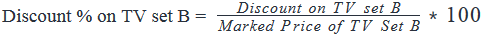• Discount on TV set B = 0.25 * Marked Price of TV set B = 0.25Q’’
• So, Q = Q’’  - 0.25Q’’ = 0.75Q’’
• So, we have P = P’’ and Q = 0.75Q’’

However, we do not know any relation between P’’ and Q’’ and between P’ and P’’ and between Q’ and Q’’

Step 4: Analyze Statement 2 independently

(2) The shopkeeper made a profit of 25 percent on set A.

• We know that Selling price of TV set A = Cost Price of TV set A + Profit made on TV set A
•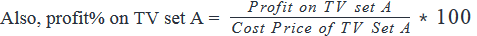• Profit on TV set A = 0.25*p’
• So, we can write P = P’ + 0.25P’ = 1.25P’
• So, using the 2 cases discussed in Step 1 and 2, we can write:
• Either Q = 0.8 *1.25P’ = P’
• Or Q = 1.2 *1.25P’ = 1.5P’

However it does not tell us anything about the profit made on TV set  B, i.e. the value of Q – Q’. Insufficient to answer.

Step 5: Analyze Both Statements Together (if needed)

• From statement-1, P = P’’ and Q = 0.75Q’’
• From statement-2, P= 1.25P’

Combining both the statements does not give us any new information to answer the question.

QUESTION: 7

If y = |x-2| + |x| - |x+2| where x is an integer, then y can take how many non-zero integral values between -10 and 10, exclusive?

Solution:

Given

• x is an integer
• y = |x-2| + |x| - |x+2|

To Find: Number of non-zero integral values of y such that -10 < y < 10

Approach

1. We see that y is an expression in modulus (‘absolute value’ is also known as modulus). The expressions inside the modulus will change the signs at their zero points.
1. For example, an expression |x-2| will have different signs for x ≥ 2 and x < 2. So, 2 will be the zero point of the expression |x-2|
2. So the expression will change signs at -2, 0 and 2. Hence, there will be 4 ranges in which we need to analyse the values of y:
1. Range-I: x < -2
2. Range-II: -2 ≤ x ≤ 0
3. Range-III: 0 < x ≤ 2
4. Range-III: x > 2

3. We will evaluate the possible values of y for each range such that -10 < y < 10

Working Out

1. Range-I: x < -2
1. |x-2| = -(x-2), as for x < -2, (x-2) < 0
2. |x| = -x, as for x < -2,  x < 0
3. |x+2| = -(x+2), as for x < -2, (x+2) < 0
4. y = -x + 2 – x + x + 2 = -x + 4. So, possible values of y between -10 and 10 = {7, 8, 9}

2. Range-II:  -2 ≤ x ≤ 0
1. |x-2| = -(x-2), as for -2 ≤ x ≤ 0, (x-2) ≤ 0
2. |x| = -x, as for -2 ≤ x ≤ 0, x ≤ 0
3. (x+2) = x+2, as for -2 ≤ x ≤ 0, (x+2) ≥ 0
4. y = - x + 2 – x – x – 2 = -3x. So, possible values of y = {3, 6}
1. We did not consider 0, as y should take only non-zero integral values.

3. Range-III: 0 < x ≤ 2
1. |x-2| = (-x-2), as for 0 < x ≤ 2, (x-2) ≤ 0
2. |x| = x, as for 0 < x ≤ 2, x > 0
3. |x+2| = x+2, as for 0 < x ≤ 2, (x+2) > 0
4. y = - x + 2 + x – x – 2 = -x. So, possible values of y = {-1, -2}

4. Range-IV: x > 2
1. |x-2| = x – 2, as for x > 2, (x-2) > 0
2. |x| = x, as for x > 2, x > 0
3. |x+2| = x+2, as for x > 2, (x+2) > 0
4. y = x – 2 + x – x -2 = x – 4. So, possible values of y = {-1, 1, 2, ……..9}

5. So, possible non-zero integral values of y = {-2, -1, 1, 2, ……9}, i.e. a total of 11 values.

QUESTION: 8

What is the remainder when the positive integer x is divided by 5?

(1) |x- 5y| = 3, where y is a positive integer

(2) |x| + 2 is divisible by 15

Solution:

Steps 1 & 2: Understand Question and Draw Inferences

Given: x is a positive integer

To Find: The remainder when x is divided by 5

Step 3: Analyze Statement 1 independently

(1) |x- 5y| = 3, where y is a positive integer

• The above inequality refers to all the values of x that are at a distance of 3 units from 5y on the number line.
• So, x = 5y + 3. Hence, x when divided by 5 will leave a remainder 3 OR
• x =  5y -3 = 5y -5 + 5 -3 = 5(y-1) + 2. Hence, x when divided by 5 will leave a remainder 2
• The equation can also be solved algebraically as below:
1. If, x – 5y ≥ 0. then |x-5y| = x-5y.
1. So, , x = 5y + 3. Hence, x when divided by 5 will leave a remainder 3
2. If x- 5y < 0, then |x-5y| = -(x-5y)
1. So, x = 5y -3 = 5(y-1) + 2. Hence, x when divided by 5, will leave a remainder 2.

Step 4: Analyze Statement 2 independently

(2) |x| + 2 is divisible by 15

|x| + 2 = 15k, where k is a positive integer

|x| = 15k -2 = 15(k-1) +13 = 15(k-1) + 10 + 3

Hence, x when divided by 5, will leave a remainder 3. Sufficient to answer.

Step 5: Analyze Both Statements Together (if needed)

Since, we have a unique answer from step 4, this step is not required.

QUESTION: 9

If x is a non-zero integer, is x prime?

(1) The number x is at a distance less than 2 units from the number 1.5 on the number line.

(2) The sum and product of roots of a quadratic equation ax2+bx+c   are 5 and 6 respectively.

Solution:

Step 1 & Step 2: Understanding the Question statement and Drawing Inferences

Given Info:

• x is a non-zero integer. This means x≠0.

To find:

• We have to determine whether x is a prime number or not.

Step 3: Analyze statement 1 independently

Statement 1:

• The number x is at a distance less than 2 units from the number 1.5 on the number line.
• We can represent the above statement on the number line.
• Representing this on number line, we get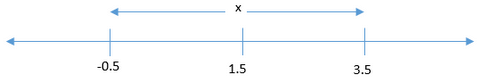• Since we are given x is an integer, the possible values of x for the above range of values of x will be → x= {0,1,2,3}
• Now, since x is also given a non-zero integer, we can reject the value of x=0
• So, this gives us x= {1,2,3}
• Now, since 1 is not a prime number and 2 & 3 are prime numbers, we cannot determine whether x will be a prime number or not.
• Hence Statement 1 is not sufficient to arrive at a unique answer.

Step 4: Analyze statement 2 independently

Statement 2:

• The sum and product of roots of a quadratic equation ax2 + bx + c are 5 and 6 respectively.
• Let the roots of the equation be p & q
• Now for a quadratic equation in x, we know
• Sum of the roots = p + q
⇒ p + q = 5 (Equation 1)
• Product of the roots = p*q
⇒p * q = 6 (Equation 2)
• Using the above two equations of p and q, we can find the values of p & q.
• Using Equation 2, we get
• p = 6/q
• Putting the above value of p in equation 1, we get
• 6/q+q=5
• Multiplying both sides by q, we get
• 6 + q2 = 5q
• Rearranging the terms, we get
• q2 - 5q + 6 = 0
• Solving the above quadratic equation for value of q
• q2 - 3q - 2q + 6 = 0
• q(q - 3) - 2(q - 3) = 0
• (q - 2)(q - 3) = 0
• Hence, q=2 or q=3
• Putting q=2 in equation 2, we get
• p * (2) = 6
• p = 3
• Putting q=3 in equation 2, we get
• p * (3) = 6
• p = 2
• Hence the possible values of p & q will be
• Either (p,q) = (3,2) or (p,q) = (2,3)
• Now these values of p & q are roots of the equation ax2+bx+c, and thus will also be the values of x, because these values of p & q will satisfy the quadratic equation in x.
• Hence we can say that, → x = 2 or 3
• Now since both 2 & 3 are prime numbers, we can say with surety that x will always be a prime number.
• Hence statement 2 is sufficient to arrive at a unique answer.

Step 5: Analyze the two statements together

• Since from statement 2, we were able to arrive at a unique answer (as shown in step 4), combining and analysing statements together is not required.
• Hence the correct answer is option B- Only statement 2 is sufficient to answer this question.
QUESTION: 10

If integers p and q are the roots of the equation ax2 + bx + c = 0, where a, b and c are constants and a > 0, by what percentage is c greater than |b|?

(1) |p+1| = |q – 3|

(2) The greatest number that divides both |p| and |q| is 2 and the smallest number that is divisible by both |p| and |q| is 12

Solution:

Steps 1 & 2: Understand Question and Draw Inferences

Given:  ax2 + bx + c = 0

• Roots are p and q
• p and q are both integers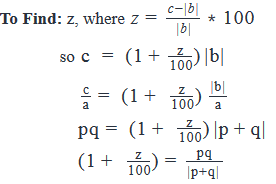Thus, to find the value of z, we need to know the values of: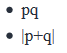Step 3: Analyze Statement 1 independently

(1) |p+1| = |q-3|

• Case 1: p + 1 = q – 3
• p – q = -4
• Linear equation with 2 unknowns. Multiple values of p and q possible
• pq and |p+q| not determined

• Case 2: p + 1 = -(q-3)
• p + q = 2
• Linear equation with 2 unknowns. Multiple values of p and q possible
• |p+q| = 2
• But pq not determined

Since unique values of pq and |p+q| have not been determined,

Not Sufficient

Step 4: Analyze Statement 2 independently

(2) The greatest number that divides both |p| and |q| is 2 and the smallest number that is divisible by both |p| and |q| is 12

• GCD (|p|, |q|) = 2
• LCM (|p|, |q|) = 12
• Possible values of (|p|, |q|) that satisfy St. 2:
• (6,4) or (4,6)
• (2,12) or (12,2)
• |p||q| = GCD * LCM = 2*12 = 24
• |pq| = 24

• Case 1 : p and q are both positive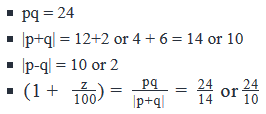• Case 2 : p positive, q negative or vice versa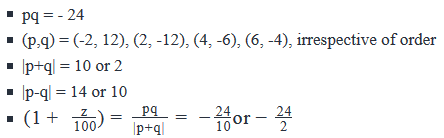• Case 3 : p and q are both negative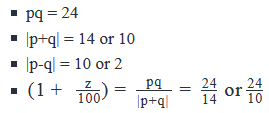2 values of z possible in Cases 1 and 3

2 different values of z possible in case 2

Not Sufficient

Step 5: Analyze Both Statements Together (if needed)

• From Statement 1
• Either p + q = 2
• Or p – q = -4
• |p-q| = 4
• In none of the 3 cases discussed in Statement 2 is |p – q| = 4
• So, the possibility of p – q = -4 is rejected
• Therefore, from Statement 1, p + q = 2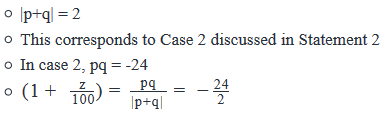From the above equation, a unique value of z will be obtained.

Sufficient.

QUESTION: 11

If |x - 5| = 2|x - 8|, then what is the value of x?

(1) |x2 – 100| > 50

(2) |x2 – 49| =0

Solution:

Steps 1 & 2: Understand Question and Draw Inferences

• |x - 5| = 2|x - 8|. The points at which the sign of the modulus(‘absolute value is also known as modulus) would change are {5, 8}.
• For example, an expression |x-2| would be positive for all values of x > 2 and non-negative for all values of x ≤ 2. So, the sign of the expression changes at x = 2
• Thus, for the above inequality there would be 2 zero points (5, 8) and hence there would be following 3 ranges :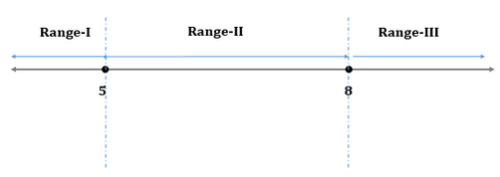• Range-I: x ≤5
• |x-5| = -(x-5), as for x ≤5, (x-5) ≤ 0
• |x-8| = -(x-8), as for x ≤5, |x-8| = x- 8
• -(x-5) = -2(x-8)
• -x + 5 = -2x + 16
• x = 11,
• x = 11 is not an acceptable solution as we have assumed x < 5.
• Thus, there is no value of x in this range that satisfies the equation |x - 5| = 2|x - 8|.

• Range-II: 5 < x <  8
• |x-5| = x-5, as for 5 < x < 8, (x-5) > 0
• |x-8| = -(x-8), as for 5 < x <  8, (x-8) < 0
• x – 5 = -2(x-8)
• x -5 = -2x + 16
• x = 7…..(2)
• x = 7 lies between 5 and 8, hence it may be an acceptable solution

• Range-III: x ≥ 8
• |x-5| = x-5, as for x ≥ 8, (x-5) > 0
• |x-8| = x-8, as for x ≥ 8, (x-8) ≥ 0
• x – 5 = 2x – 16
• x = 11
• x = 11 is greater than 8, hence it may be an acceptable solution

To Find: Unique value of x

• From our analysis above, we’ve seen that x = {7, 11}

Step 3: Analyze Statement 1 independently

(1) |x2 – 100| > 50

• We’ve already deduced in Steps 1 and 2 that x = {7, 11}
• Putting x = 7 in the inequality, we have |49 – 100| = 51 > 50. Thus x = 7 is an acceptable solution. Let’s see if this is the only solution.
• Putting x = 11 in the inequality, we have |121-100| = 21 < 50. Thus x = 11 is not an acceptable solution.

Hence, x = 7. Sufficient to answer.

Step 4: Analyze Statement 2 independently

(2) |x2 – 49| =0

• We’ve already deduced in Steps 1 and 2 that x = {7, 11}
• Putting x = 7 in the equation, we have |49 – 49| = 0. Thus x = 7 is an acceptable solution.
• Putting x = 11 in the equation, we have |121- 49| ≠ 0. Thus x = 11 is not an acceptable solution.

Hence x = 7. Sufficient to answer.

Step 5: Analyze Both Statements Together (if needed)

As we have a unique answer from steps 3 and 4, this step is not required.

QUESTION: 12

|x + 3| = |y -4|, where x and y are non-zero integers. If |x| < 5 and |y| < 5, what is the maximum possible value of -|xy|?

Solution:

Given

• x, y are integers such that x, y ≠ 0
• |x + 3| = |y -4|
• |x|, |y| < 5

To Find: Max(-|xy|)

Approach

1. As |xy| ≥ 0, -|xy| ≤ 0
2. So, to maximize the value of -|xy|, we need to minimize the value of |xy|. This is because, smaller the magnitude of a negative number, larger is the value of that negative number.
For example, -2 > -4 > -5, > -100….​
1. Note that |xy| ≠ 0, as x, y ≠ 0
3. As we are given a relation in x and y and we know that |x|, |y| < 5, we will use them to find out the possible values of x and y and hence the minimum value of |xy|

Working Out

1. As |x| < 5, ​
1. The above inequality refers to all the values of x that are at a distance of less than 5 units from 0 on the number line​​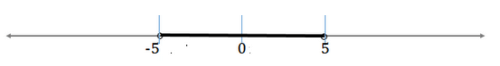2. -5 < x < 5.
3. So, x can take values of {-4, -3,,,-1, 1, 2, ,,,4}
2. Similarly, as |y| < 5, -5 < y < 5
1. So, y can take values of {-4, -3,….-1, 1, 2, ……4}
3. |x + 3| = |y -4|
1. We can interpret it as: (the distance of x from -3) = (distance of y from 4)
2. The value of |xy| will be minimum, when values of x and y are as close to zero as possible.
3. As -5 < x, y < 5 and we need to look for values of x that greater than -3 and values of y that are less than 4 and satisfy the constraint in 3a
4. We can see that the values for which |xy| is minimum is satisfied by
1. x = -1 and y = 2. In this case |xy| = 2 OR
2. x = 2 and y = -1. In this case |xy| = 2
4. So, maximum value of -|xy| = -2

QUESTION: 13

If |x + 4| = 8, what is the sum of all the possible values of x?

Solution:

Given Info:

• |x + 4| = 8
• The above absolute value means that x is at a distance of 8 units from number -4 on the number line.
• Plotting this on number line, we have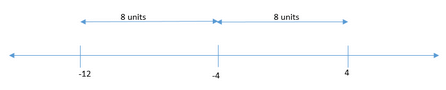• This means x = -12 or x = 4

To find:
Sum of all possible values of x

Approach:

• We have already plotted the different possible values of x on the number line.
• By knowing the various possible values of x, we will add all the values to get the sum of all values of x.

Working out

•  From the number line we will get either x=-12 or x=4
• Sum of the all values of x will be
• -12+4 = -8

• The sum of all possible values of x will be -8. Hence option C is correct.
QUESTION: 14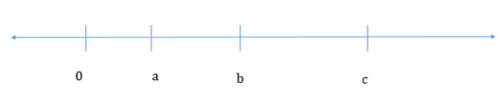On the number line shown, the distance between 0 and a, a and b and a and c is in the ratio of 1:2:3. If the distance of point b from 15 is twice the distance of point a from 15, what is the value of |c|?

Solution:

Given :

• Points a, b and c such that c > b > a
• a: (b – a) : (c – a) = 1: 2: 3
• |b – 15| = 2 * |a-15| (We are taking the absolute values here, as we do not know the position of 15 with respect to a and b. For example, if b > 15, then |b – 15| = b – 15 but if b < 15, then |b – 15| = 15 - b)

To Find: value of |c|

• That is, Distance of c from 0, i.e.  the coordinate of point C

Approach

1) For finding the coordinates of point c, we need to find the coordinates of a, because we are given a relation between the coordinates of point a and point c by a:(c-a) = 1:3

2) Let the distance of point a from 0 be x, i.e. the coordinate of point a is x.

• As a: (b-a) = 1: 2, we have, So ,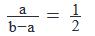• Similarly, as a: (c – a) = 1: 3 , we can write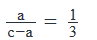• We can use the above relation to find the coordinates of points b and c in terms of x.

3) Now, we are given the relationship between the distance of points a and b with respect to 15. However, we do not know the position of 15 with respect to a and b on the number line. So,  the following cases can occur depending upon the position of 15 on the number line:

• 15 ≤ a < b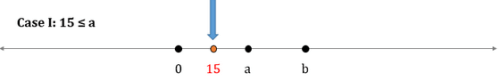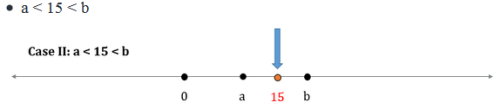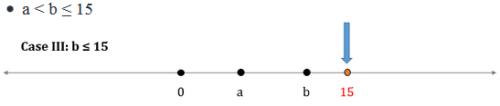4)  As we have calculated the coordinates of points b, c in terms of x in Step-1, we will evaluate the above cases along with the relation |b – 15| = 2 * |a-15|  to arrive at a unique value of x.

5)  Once we calculate the value of x, we can calculate the coordinate of point c.

Working Out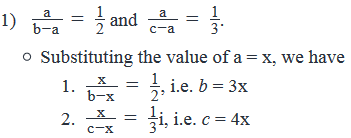2)  Case-I: 15 ≤ a

• Distance between 15 and a = a – 15 = x – 15 ( as a is greater than 15)
• Distance between 15 and b = b – 15 = 3x – 15 ( as b is greater than 15)
• 3x – 15 = 2*(x – 15) {as |b – 15| = 2 * |a-15| }
• 3x – 15 = 2x – 30
• x = -15, which is not possible as x is the coordinate of a point that lies on the right hand side of 0, i.e. it is positive. So, x > 0.
• Thus, we can reject this case.

3)  Case-II: a < 15 < b

• Distance between 15 and a = 15 – a = 15 – x  (as 15 is greater than a)
• Distance between 15 and b = b – 15 = 3x – 15 (as b is greater than 15)
• 3x – 15 = 2*(15 – x) {as |b – 15| = 2 * |a-15| }
• 3x – 15 = 30 – 2x
• 5x = 45, i.e. x = 9.
• In this case we have assumed that a < 15. So, x < 15 ( As the coordinate of point a is x). Also x > 0, since it is the coordinate of a point that lies on the right hand side of 0.
• So, 0 < x< 15. As we have calculated x = 9, this is a possible case
• We have calculated in step-1, the coordinate of point c = 4x. So, we have 4x = 36

4)  Case-III: b ≤ 15

• Distance between 15 and a = 15 – a = 15 – x  (as 15 is greater than a)
• Distance between 15 and b = 15 – b = 15 – 3x  (as 15 is greater than b)
• 15 – 3x = 2*( 15 – x) {as |b – 15| = 2 * |a-15| }
• 15 – 3x = 30 – 2x, i.e. x = -15,
• We know that x > 0. So, x = -9 is not a valid value.
• Thus, we can reject this case.

So, the only possible value of x = 9, for which we have the coordinate of point c = 4x = 36.

Thus |c| = 36.

QUESTION: 15

What is the value of integer x?

(1) x + x2 = 0

(2) |x| + x2 = 2x

Solution:

Steps 1 & 2: Understand Question and Draw Inferences

• x is an integer

To Find: Unique value of x

Step 3: Analyze Statement 1 independently

(1) x + x2 = 0

x(1+x) = 0

x = 0 or x = -1

Since we do not have a unique value of x, the statement is insufficient to answer.

Step 4: Analyze Statement 2 independently

(2) |x| + x2 = 2x

• Following cases can occur:
• If x ≥ 0
• Then, |x| = x
• So, the equation in Statement 2 becomes:
• x + x2 = 2x
• x2 - x = 0
• x(x – 1) = 0
• Either x = 0 or 1. Both values satisfy the condition in this case (that x ≥ 0). So, both these values are possible
• If x < 0
• Then, |x| = -x
• So, the equation in Statement 2 becomes:-x + x2 = 2x
• x2 - 3x = 0
• x(x – 3) = 0
• Either x = 0 or 3. Both the values are rejected as we have assumed x < 0

So, x = 0 or 1. Insufficient to answer.

Alternate Method

As |x| and x2 is always ≥ 0 (irrespective of the value of x), |x| + x2≥ 0. Thus, the equation in statement 2 tells us that 2x ≥ 0.

So, x will never be less than zero. Hence, we can assume |x| = x and solve for the values of x.

As shown above, we'll get: x = 0 or 1.

Step 5: Analyze Both Statements Together (if needed)

1. From statement 1, we inferred that: x = 0 or -1
2. From statement 2, we inferred that: x = 0 or 1

Combining both the statements, we have x = 0. Sufficient to answer.

QUESTION: 16

The positive integer y is at a distance of 2 units from the nearest multiple of a single digit positive integer x. What is the minimum positive value that should be added to y, so that it becomes divisible by x?

(1) y is x/2  units more than the square of x, where x/2  is even

(2) y – 3 is a multiple of 5

Solution:

Steps 1 & 2: Understand Question and Draw Inferences

• x is an integer such that 0 < x < 10
• y is an integer > 0
• Let the multiple of x be mx.
• So, two values are possible for values of y:
• y = mx + 2 or
• y = mx – 2
• Possible values of x: {1….9}
• However, as y is 2 units away from a multiple of x,
• x ≠ 1(because then y will be a multiple of x)
• x ≠ 2(because then y will be at a maximum distance of 1 unit from a multiple of x)
• x ≠ 3(because then y will be at a maximum distance of 1 unit from the nearest multiple of x)
• So, x = {4, 5, ….9}………………(1)

To Find: How much should be added to y so that it is divisible by x?

• Case-I: If y = mx – 2, then 2 should be added to y to get to make it divisible by x. The resulting number will be mx
• Case-II:If y = mx + 2, then to represent y as the next multiple of x i.e. (m+1)x, we should add, (m+1)x – (mx +2) = mx + x – mx – 2= x-2. So, x-2 should be added to y= mx + 2 to make y divisible by x.
• So, the statement will be sufficient if:
• Both the cases give us the same answer OR
• We are able to reject 1 case and the other case gives us a unique answer.

Step 3: Analyze Statement 1 independently

(1) y is x/2 units more than the square of x, where x/2 is even

• Case-I: y = mx + 2.
• As per Statement 1, y=x2+(x/2)
• So, we need to add   to y to make it divisible by x.
• As x/2  is an even integer, x/2={2,4} . Any value of x/2, would result in value of x ≥ 10. Since 0 < x < 10, these values cannot be accepted.
• So, x = {4, 8}
• For x = 4, we have y =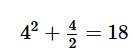), which is of the form 4x + 2. So, x = 4 is a possible value of x
• For x = 8, we have y =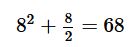. However, 68 is of the form 8x + 4 and not of the form 8x + 2. So, x = 8 is not a possible value of x.
• So, we have x/2=2
• Hence 2 needs to be added to y to make it divisible by x

• Case-II: y = mx – 2
• 2 should be added to y so that it is divisible by x

As we have a unique answer from both the cases, the statement is sufficient to answer.

Step 4: Analyze Statement 2 independently

(2) y – 3 is a multiple of 5

• Case-I: y = mx + 2
• As per statement 2, y -3 = 5k, where k is an integer > 0
• So, mx + 2 -3 = 5k
•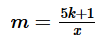• For m to be an integer, 5k + 1 should be a multiple of x. Let’s observe a few cases and see if this constraint gives us a unique value of x-2: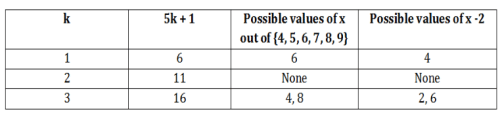•  This case does not give us a unique value of x -2.

Case-II: y = mx – 2

• 2 should be added to y so that it is divisible by x.

As the cases do not give us a unique value of the number that should be added to make y divisible by x, this statement is not sufficient to answer.

Step 5: Analyze Both Statements Together (if needed)

As we have a unique answer from step-3, this step is not required.

QUESTION: 17

If x is an integer, is x2 > 25?

(1) |x – 3| > 5

(2) (x + y)2 > 49, where y is an integer such that |y| < 2

Solution:

Steps 1 & 2: Understand Question and Draw Inferences

• x is an integer

To Find : Is x2 > 25

• That is, Is x2-25>0 or (x + 5) *( x - 5 ) > 0 ?
• Let’s find the range of x using the wavy line method.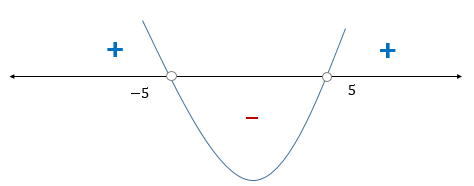• The inequality is positive for x > 5 or x < -5.
• Hence, the answer to the question, Is x2 > 25 will be YES  if
• x > 5 or x < -5
• The answer to the question, Is x2 > 25 will be NO if
• -5 ≤ x ≤ 5

Step 3: Analyze Statement 1 independently

(1) |x – 3| > 5

• The above inequality refers to all the values of x that are at a distance of greater than 5 units from 3 on the number line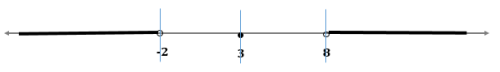• It can also be solved algebraically as below:
• If x – 3 > 0,
• x – 3 > 5, i.e. x > 8
• In this case x > 5
• If x – 3 < 0,
• -x + 3 > 5, i.e. x < -2.
• In this case x is less -2,, but we needed x < -5
• As we do not have a unique answer, the statement is insufficient to answer.

Step 4: Analyze Statement 2 independently

(2) (x + y)2 > 49, where y is an integer such that |y| < 2

• Evaluating the range of y:
• As |y| < 2, we have -2 < y < 2
• Evaluating the range of x
• (x+y)2 - 72 > 0
• (x + y + 7)(x + y -7) > 0
• Now the product of two terms can be positive if both the terms are positive or both the terms are negative. So, following cases can occur:
• Case-I: (x+y+7) > 0 and (x+y-7) > 0
• This can be simplified to x + y > -7 and x + y > 7
• Combining both, we can say that
• x + y > 7
• x>7-y
• As maximum value of y can be just less than 2, x > 7 – 2 = 5. So, x > 5
• Case-II: (x+y+7) < 0 and (x+y-7)< 0
• This can be simplified to (x+y) < -7 and (x+y) < 7
• Combining both, we can say that x+ y < -7
• x < -7 –y
• As minimum value of y will be just greater than -2, x < -7 +2 = -5. So, x < -5

As in both the cases, x > 5 or x < -5, the answer to the question is YES.

Hence, the statement 2 alone is sufficient to answer.

Step 5: Analyze Both Statements Together (if needed)

As we have a unique answer from step 4, this step is not required.

QUESTION: 18

If z is an integer such that ||z - 30| - 43| = 62 which of the following could be value of |r|, where r is the remainder obtained when z is divided by 7?

I. 0

II. 2

III. 4

Solution:

Given:

• Integer z
• ||z-30|-43|=62
• When z is divided by 7, the remainder is r
• So, z = 7k + r, where k is an integer

To find: Can |r| be {0, 2, 4}?

Approach:

• Since the remainder is always non-negative, |r| = r. So, the question is: Can r be {0, 2, 4}?
• To answer this question, we first need to find the possible values for z.

Working Out:

• Simplifying the given expression for z
• ||z-30|-43|=62
• Case 1: |z – 30| - 43 = +36
• So, |z-30| = 79
• Either z – 30 = 79
• So, z = 109
• Remainder when z is divided by 7 is 4
• Or z – 30 = - 79
• So, z = -49
• Remainder when z is divided by 7 is 0
• Case 2: |z – 30| - 43 = -36
• So, |z – 30| = 7
• Either z – 30 = 7
• So, z = 37
• Remainder when z is divided by 7 is 2
• Or z – 30 = -7
• So, z = 23
• Remainder when z is divided by 7 is 2
• Notice here that that we got 4 possible values of z : -49, 23, 37, 109
• Evaluating the 3 options
• We’ve seen above that the possible values of r when z divided by 7 are: {0, 2, 4}
• So, Options I, II and  III are possible

Looking at the answer choices, we see that the correct answer is Option D

QUESTION: 19

List A = {-x, x, |x|, x2, -6, 6}

If x is a non-zero integer such that  -5 ≤ x ≤ 5, the ratio of the range of the list A to the median of the list A must lie between which of the following?

Solution:

Given

• List A =  {-x, x, |x|, x2, -6, 6}
• x is an integer such that -5 ≤ x ≤ 5 and x ≠ 0

To Find: Minimum and Maximum value of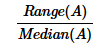Approach

1. To calculate the minimum and maximum values ofwe need to find the minimum and the maximum values of the range and the median of list A.
2. To calculate the range and the median, we need to first order the list.
1. In the list, the terms |x|, x2 and 6 is always positive. So, the smallest term of the list = { -x, x, or -6}
2. Since x can have a minimum value of -5 and –x can also have a minimum value of -5, the smallest term of the list = -6,
3. The largest two terms = x2 or 6 depending upon the value of x
4. That leaves us with 3 terms in the middle: -x, x, and |x|. Since the magnitude of all these terms are equal, their positioning in the ordered list will depend on the sign of x.
5. Hence the median will depend on the sign of x. So, following cases are possible:
6. Case-I: If x < 0
• The ordered list= { -6, x, -x, |x|, 6 or x2, x2 or 6}. The last two terms will be 6 or x2 depending on the value of x.  For example, x>6, if |x| > 2. So, for x = { -5, -4, -3, }, x2 >6 else for x = {-2, -1, }, x2 <6. However, in both the cases, the 3rd and the 4th terms will be –x and |x|. Please note that as x < 0, |x| = -x. So, in this case |x| = -x, i.e the 3rd an 4th term will be equal.
1. So, Median =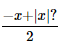. So, median  =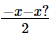=−x  . As x < 0, the median i.e. –x will be positive.
2. The Range(A) = Largest term – Smallest term
1. Smallest term = -6
2. Largest term =   , if |x| > 2 else it will be 6
3. So, Range(A) =
1.  ( x2  - (-6)) = x+6 or
2. 6 – (-6)) = 12
7.   Case-II: If x > 0

1. The ordered list = { -6, -x, x, |x|, 6 or x2, x2 or 6}. The last two terms will be 6 or x2 depending on the value of x. For example,x2 > 6 if |x| > 2. So, for x = { 3, 4, 5}, x2 > 6, else for x = {1, 2}, x2 < 6. However, in both the cases, the 3rd and the 4th terms will be x and |x|.As we have assumed that x > 0, |x| = x, i.e. the 3rd and 4th terms will be equal.
2. So, Median =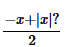. As x we have assumed x > 0, |x| = x. So, median =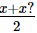=x.  As x >0, the median i.e. x will be positive.
3. The Range(A) = Largest term – Smallest term
1. Smallest term = -6
2. Largest term = x2, if |x| > 2 else it will be 6
3. So, Range(A) =
1.  ( x2  - (-6)) = x+6 or
2.  6 – (-6)) = 12
8. Also, we can observe here the value of median in both the cases:
1. Median = -x, if x < 0 and
2. Median = x , if x > 0
3. This is the property of |x|. So, we can say that the median = |x|
3. Since Median(A) = |x|, we need to find the value of Median(A) for each value of |x| to calculate the maximum and minimum values of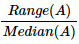Also, since the value of x2 is same for x and –x, we do not need to calculate the value of Range(A) all values of x.Since the range of x is symmetric about 0, for all the negative values of x, there will be corresponding positive value of x with the same magnitude. So, we need to find the range for unique values |x| only, i.e. |x| = {1,2,3,4,5}
1. For example, for x = 1 and x= -1, the Range(A) will remain the same. So, we can just calculate the range for |x| = 1

Working Out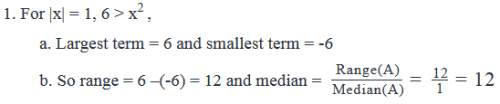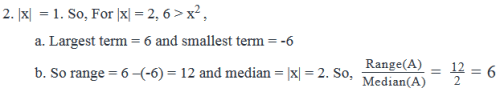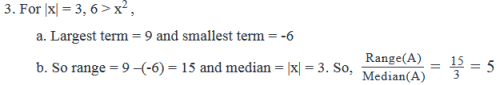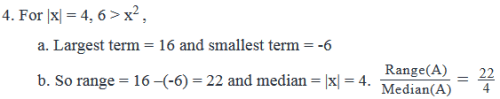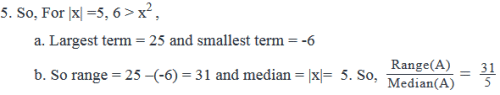Maximum value of=12 and minimum value of=5

Hence, the ratio of the range of the list A to the median of the list A must lie between 5 and 12.

QUESTION: 20

If P & Q are integers such that |P|Q=4 , is P negative?

(1) |P|+|Q|=|P+Q|

(2) P2−5|P|+4=0

Solution:

Step 1 & Step 2: Understanding the Question statement and Drawing Inferences

Given Info:

• P & Q are given as integers. Also, |P|Q=4
• The number ‘4’ in the right hand side of the equation can be expressed in exponential form using the following integers as bases: 2, -2, 4 or -4.
• Now comparing the bases obtained from right hand side of the equation to the base → |P| on the left hand side of the equation we will be left with,
• Case 1: |P|=2
• Now |P|=2, means that integer P is at a distance of 2 units from number 0 on the number line.
• Thus P = 2 or -2
• Case 2: |P|=4
• Now |P|=4, means that integer P is at a distance of 4 units from number 0 on the number line.
• Thus P = 4 or -4
• The cases when |P|=-2 and |P|=-4 can be rejected as absolute value of a number cannot be negative.
• So from the above 2 cases, we will have P = {-4,-2,2,4}.

To find: Is P negative?

• From the 2 cases above, we have P to be either positive or negative. So, we won’t be able to tell whether P is negative or not. Hence, we need to analyze the given statements further to see if P is negative or not.

Step 3: Analyze statement 1 independently

Statement 1:

• |P|+|Q|=|P+Q|
• From the analysis above, we have P = {-4,-2,2,4}

• Case a: P=-2,
• When P=-2 → We will have |P|=2, This will give Q=2, as
• When Q=2, we will have |Q| = 2
• Left hand side of the equation → |P|+|Q|=2 + 2 = 4
• Right Hand side of the equation → |P+Q|=|-2+2| = 0
• Now since, 4≠0 ⇒ |P|+|Q|≠|P+Q|
• The statement |P|+|Q|=|P+Q| will not hold true when P=-2

• Case b: P=2,
• When P=2 → We will have |P|=2, This will give Q=2, as
• When Q=2, we will have |Q| = 2
• Left hand side of the equation → |P|+|Q|=2 + 2 = 4
• Right Hand side of the equation → |P+Q|=|2+2| = 4
• Now since, 4=4 ⇒ |P|+|Q|=|P+Q|
• The statement |P|+|Q|=|P+Q| will hold true when P=2

• Case c: P=4,
• When P=4 → We will have |P|=4, This will give Q=1, as
• When Q=1, we will have |Q| = 1
• Left hand side of the equation → |P|+|Q|=4 + 1 = 5
• Right Hand side of the equation → |P+Q|=|4+1| = 5
• Now since, 5=5 ⇒ |P|+|Q|=|P+Q|
• The statement |P|+|Q|=|P+Q| will hold true when P=4

• Case d: P=-4,
• When P=-4 → We will have |P|=4, This will give Q=1, as
• When Q=1, we will have |Q| = 1
• Left hand side of the equation → |P|+|Q|=4 + 1 = 5
• Right Hand side of the equation → |P+Q|=|-4+1| = 3
• Now since, 5≠3 ⇒ |P|+|Q|≠|P+Q|
• The statement |P|+|Q|=|P+Q| will not hold true when P=-4
• So the values of P, which satisfy the first statement will be either 2 or 4.
• From this, we can tell definitely that P is not negative.
• Hence statement 1 is sufficient to arrive at a unique answer.

Step 4: Analyze statement 2 independently

Statement 2:

• P2−5|P|+4=0
• Now, since  is always positive, we will write  in the above equation as | , to make a quadratic equation in |P|.
• This will result in no change in the quadratic equation as  is essentially same as  for the equation because  is also always positive.
• Solving, |P|2−5|P|+4=0
• |P|2 - 5P + 4 = 0
• |P|2 - 4|P| - |P| + 4 = 0
• |P|(|P| - 4) - 1(|P| - 4) + 4 = 0
• (|P| - 1)(|P| - 4) = 0
• |P| = 1 or |P| = 4
• Now, |P|=1, means that integer P is at a distance of 1 unit from number 0 on the number line.
• Thus P = {-1,1}
• These values of P can be rejected, as P can only have values {-4,-2,2,4}, as established above.
• Now, |P|=4, means that integer P is at a distance of 4 units from number 0 on the number line.
• Thus, P = {-4,4}
• Out of this we have P=4 and P=-4 as the only possible solutions.
• We get the value of P as 4 or -4 from the second statement.
• Thus, we cannot tell whether P will be negative or not.
• Hence Statement 2 is not sufficient to arrive at a unique answer.

Step 5: Analyze the two statements together

• Since from statement 1 we are able to arrive at a unique answer (as shown in step 3) combining and analysing both statements together is not required.
• Hence the correct answer is option A- Only statements 1 is sufficient to arrive at a unique answer.
QUESTION: 21

If m is a negative integer and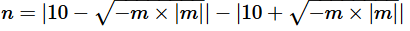, then n can take how many positive values?

Solution:

Given:

• m is a negative integer
•To Find: No. of positive values of n

Working Out

Assume

• y =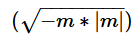Therefore

• n = |10-y| - |10+ y|

n will be positive if

•  |10-y| >  |10+ y|

Since m is a negative integer,

• -m will be a positive integer

Therefore,

•  -m * |m| will be positive.

The square root of any positive number is positive (the square root of a negative number is not defined). So,

• y =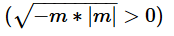Since y is a positive number, 10 + y will be positive for all possible values of y. So the inequality can be simplified as:

• |10 - y| > 10 + y
• Now, the magnitude of 10 minus a positive number cannot be greater than the sum of 10 and that positive number
• Thus, there is no instance where |10 - y| is greater than 10 + y
• So, no positive value of n is possible

QUESTION: 22

If x > 0, how many integer values of (x, y) will satisfy the equation 5x + 4|y| = 55?

Solution:

5x + 4|y| = 55
The equation can be rewritten as 4|y| = 55 - 5x.
Because |y| is non-negative, 4|y| will be non-negative. Therefore, (55 - 5x) cannot take negative values.

Because x and y are integers, 4|y| will be a multiple of 4.
Therefore, (55 - 5x) will also be a multiple of 4.
55 is a multiple of 5. 5x is a multiple of 5 for integer x. So, 55 - 5x will always be a multiple of 5 for any integer value of x.
So, 55 - 5x will be a multiple of 4 and 5.
i.e., 55 - 5x will be a multiple of 20.

Integer values of x > 0 that will satisfy the condition that (55 - 5x) is a multiple of 20:
1. x = 3, 55 - 5x = 55 - 15 = 40.
2. x = 7, 55 - 5x = 55 - 35 = 20
3. x = 11, 55 - 5x = 55 - 55 = 0.
When x = 15, (55 - 5x) = (55 - 75) = -20. Because (55 - 5x) has to non-negative x = 15 or values greater than 15 are not possible.
So, x can take only 3 values viz., 3, 7, and 11.

We have 3 possible values for 55 - 5x. So, we will have these 3 values possible for 4|y|.
Possibility 1: 4|y| = 40 or |y| = 10. So, y = 10 or -10.
Possibility 2: 4|y| = 20 or |y| = 5. So, y = 5 or -5.
Possibility 3: 4|y| = 0 or |y| = 0. So, y = 0.

Number of values possible for y = 5.

The correct choice is (C) and the correct answer is 5.

QUESTION: 23

If p > 0, and x2 - 11x + p = 0 has integer roots, how many integer values can 'p' take?

Solution:

Step 1:
The given quadratic equation is x2 - 11x + p = 0.
The sum of the roots of this quadratic equation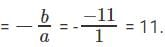The product of the roots of this quadratic equation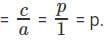Step 2:
The question states that p > 0.
'p' is the product of the roots of this quadratic equation. So, the product of the two roots is positive.
The product of two numbers is positive if either both the numbers are positive or both the numbers are negative.
We also know that the sum of the roots = 11, which is positive.
The sum of two negative numbers cannot be positive.
So, both the roots have to be positive.
Step 3:
We also know that the roots are integers.
So, we have to find different ways of expressing 11 as a sum of two positive integers.
Possibility 1: (1, 10)
Possibility 2: (2, 9)
Possibility 3: (3, 8)
Possibility 4: (4, 7)
Possibility 5: (5, 6)

Each of these pairs, will result in a different value for p.
So, p can take 5 different values.

The correct choice is (C) and the correct answer is 5.

QUESTION: 24

How many real solutions exist for the equation x2 – 11|x| - 60 = 0?

Solution:

Step 1:

Assign y = |x| and solve for y

Let |x| = y.
We can rewrite the equation x2 - 11|x| - 60 = 0 as y2 - 11y - 60 = 0
The equation can be factorized as y2 - 15y + 4y - 60 = 0
(y - 15) (y + 4) = 0
The values of y that satisfy the equation are y = 15 or y = -4.
Step 2:
We have assigned y = |x|
|x| is always a non-negative number.
So, |x| cannot be -4.
|x| can take only one value = 15.
If |x| = 15, x = 15 or -15.

The number of real solutions that exist for x2 – 11|x| - 60 = 0 is 2.

The correct choice is (B) and the correct answer is 2.

QUESTION: 25

If the curve described by the equation y = x2 + bx + c cuts the x-axis at -4 and y axis at 4, at which other point does it cut the x-axis?

Solution:

Step 1:
y = x2 + bx + c is a quadratic equation and the equation represents a parabola.
The curve cuts the y axis at 4.
The x coordinate of the point where it cuts the y axis = 0.
Therefore, (0, 4) is a point on the curve and will satisfy the equation.
Substitute y = 4 and x = 0 in the quadratic equation: 4 = 02 + b(0) + c
Or c = 4.
Step 2:
The product of the roots of a quadratic equation is c/a
In this question, the product of the roots = 4/1
Step 3:
The roots of a quadratic equation are the points where the curve (parabola) cuts the x-axis.
The question states that one of the points where the curve cuts the x-axis is -4.
So, -4 is one of roots of the quadratic equation.
Let the second root of the quadratic equation be r2.
From step 2, we know that the product of the roots of this quadratic equation is 4.
So, -4 * r2 = 4
or r2 = -1.

The second root is the second point where the curve cuts the x-axis, which is -1.

The correct choice is (A) and the correct answer is -1.Use Code STAYHOME200 and get INR 200 additional OFF Use Coupon Code